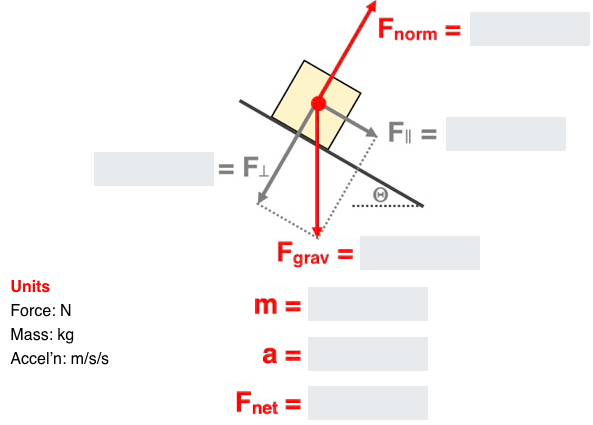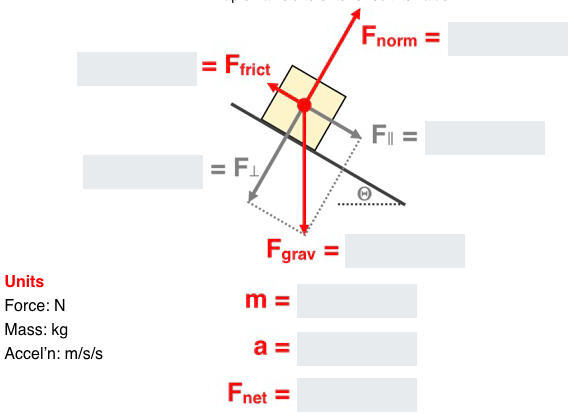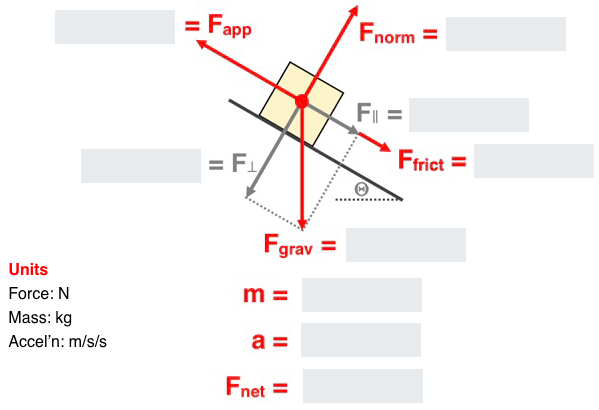## Solve It! - Inclined Planes

The Solve It! - Inclined Planes Concept Builder includes three groups of two questions spread aross three difficulty levels. Each question requires the analysis of an object accelerating along an inclined plane. The analysis involves the use of vector resolution (to determine the components of the gravity force) and Newton's second law equation.

The three difficulty levels are differentiated as follows:

• Apprentice Difficulty Level Questions 1-2: Object accelerates down the inclined plane. No friction. The mass and the incline angle are given.
• Master DifficultyLevel Questions 3-4: Object accelerates down the inclined plane. The mass, the incline angle, and the coefficient of friction are given.
• Wizard Difficulty Level Questions 5-6: Object accelerates up the inclined plane under the influence of an applied force. The force value, the mass, the incline angle, and the coefficient of friction are given.

The Physics Classroom grants teachers and other users the right to print these questions for private use. Users are also granted the right to copy the text and modify it for their own use. However, this document nor its graphics should not be uploaded to other servers for distribution to and/or display by others. The Physics Classroom website should remain the only website or server from which the document  and its graphics is distributed or displayed. We also provide a PDF that teachers can use under the same conditions. We have included a link to the PDF near the bottom of this page.

#### Solve It! - Inclined Planes

NOTE: Numerical values used in this Concept Builder are randomly generated and likely different than those published here.

Apprentice Difficulty Level
Question 1
Analyze this: A 3.68-kg object accelerates down a friction-free incline that is inclined at 19.2° above the horizontal. Complete the diagram.Question 2
Analyze this: A 8.98-kg object accelerates down a friction-free incline that is inclined at 24.7° above the horizontal. Complete the diagram.Master Difficulty Level
Question 3
Analyze this:  A 4.92-kg object slides down an inclined plane that makes an angle of 19.2° with the horizontal.The coefficient of friction is 0.215. Complete the diagram.Question 4
Analyze this:  A 14.1-kg object slides down an inclined plane that makes an angle of 26.4° with the horizontal.The coefficient of friction is 0.293. Complete the diagram.Wizard Difficulty Level
Question 5
Analyze this:  A 173-N force is applied parallel to an inclined plane to accelerate a 18.0-kg object up the incline. The coefficient of friction is 0.221. The incline angle is 15.9°. Complete the diagram.Question 6
Analyze this:  A 263-N force is applied parallel to an inclined plane to accelerate a 22.5-kg object up the incline. The coefficient of friction is 0.287. The incline angle is 21.3°. Complete the diagram.View:  Questions in PDF Format

Visit: Concept Builder || Teacher Notes || Directions